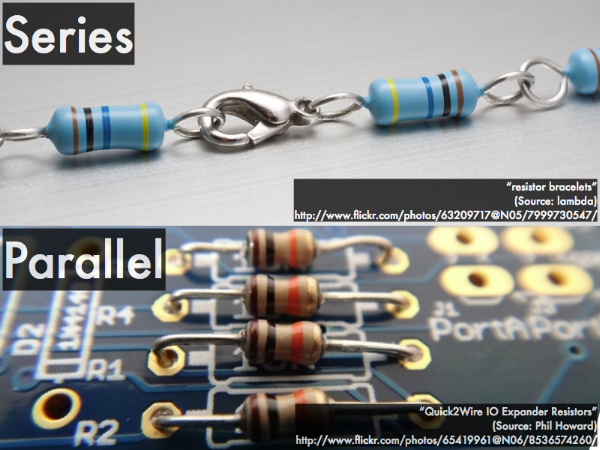## 20180314

### Online reading assignment: circuit analysis

Physics 205B, spring semester 2018
Cuesta College, San Luis Obispo, CA

Students have a bi-weekly online reading assignment (hosted by SurveyMonkey.com), where they answer questions based on reading their textbook, material covered in previous lectures, opinion questions, and/or asking (anonymous) questions or making (anonymous) comments. Full credit is given for completing the online reading assignment before next week's lecture, regardless if whether their answers are correct/incorrect. Selected results/questions/comments are addressed by the instructor at the start of the following lecture.

The following questions were asked on reading textbook chapters and previewing presentations on circuit analysis.Selected/edited responses are given below.

Describe what you understand from the assigned textbook reading or presentation preview. Your description (2-3 sentences) should specifically demonstrate your level of understanding.
"The difference between resistors running in parallel and in series; and the Kirchhoff junction rule saying that for currents, what goes in must come out."

"In addition to stacking resistors, they can also be wired in parallel so as to receive the same amount of voltage each as the original source. To add resistors in parallel, add together the inverse value of each resistor."

"That a series is where resistors are arranged so that a current may travel through them in a sequence. I also understand that a parallel circuit is arranged so that a current can travel through multiple resistors at once."

"That in a junction, charge going in equals charge going out. For a loop, the sum of voltage changes rising equals the sum of voltage changes dropping. Equivalent resistances in a series is merely the sum of each resistor whereas parallel resistors require the addition of the inverse values of each resistor added and then the sum is inversed."

"The sign (+ or -) determine if we are gaining or losing electric potential. Potential increases if going from - to + but decreases if we go from + to -."

"Whatever flows into something must come back out. Current leaving a junction must add up to what entered it."

Describe what you found confusing from the assigned textbook reading or presentation preview. Your description (2-3 sentences) should specifically identify the concept(s) that you do not understand.
"Determining what happens to current and voltage through an ideal wire."

"The loop rule seems tedious."

"I don't really understand why voltage potential difference drops and electric potential will decrease when the circuit is moving from positive to negative. I also don't understand why voltage potential difference and electric potential will increase when the circuit is moving from negative to positive. I really just don't get why it changes in the first place."

"I'd like clarification on the loop rule. I understand rise in voltage must equal drop in voltage, but I am having trouble conceptualizing the process."

"emf rise and drops and how to determine if the current or voltage goes up and down."

"I don't get why Benjamin Franklin didn't have the current flow follow the electrons it would be way simpler then! Otherwise the concepts don't seem too confusing."

"Nothing confusing yet."

"This didn't have anything that seems confusing."

"The assigned textbook reading was not really confusing but I am definitely looking forward to do some practice in class."

Determine what happens to the following parameters as current flows through an ideal wire.
(Only correct responses shown.)
Current: remains the same [81%]
Voltage: remains the same [63%]Determine what happens to the following parameters if you go through a resistor along the direction of current.
(Only correct responses shown.)
Current: remains the same [63%]
Voltage: decreases [70%]Determine what happens to the following parameters if you follow a path (regardless of current direction) into the (–) terminal and out of the (+) terminal of an ideal battery.
(Only correct responses shown.)
Current: remains the same [%56]
Voltage: increases [52%]

Briefly explain what quantity is conserved when applying Kirchhoff's junction rule.
"When applying the Kirchhoff's junction rule the current (amperage) is conserved."

"The sum of the current leaving the ideal battery is the same as the current entering the ideal battery."

"Charge flow per time."

"Not sure."

"I have no idea."

Briefly explain what quantity is conserved when applying Kirchhoff's junction rule.
"When applying the Kirchhoff's loop rule the electric potential is conserved. The initial voltage drops through all the components of the circuit."

"Electric potential energy because energy can not be lost or gain through any means in a closed system."

"Electric potential energy per charge."

"Energy is conserved."

"The sum of all voltages must be equal to zero in a closed system."

"Not sure."

"?"

Ask the instructor an anonymous question, or make a comment. Selected questions/comments may be discussed in class.
"Is there any way to calculate the resistance of something without using a multimeter directly?" (Yes, if you use the multimeter to "indirectly" measure the amount of current flowing through it, and the potential difference (voltage drop) across it, then you can use Ohm's law to calculate its resistance.)

"What reason would someone use a parallel or series resistance? Which devices or requirements would implement each in technology or other uses?" (Everything that you plug into an electrical outlet is in parallel with each other: so each can receive the same 120 V and turn on/off independently of each other. Typically control devices such as dimmers are wired in series, such that what happens to one part (by changing its resistance) will affect the amount of current flowing through the rest of the circuit (say, to a light bulb or a heating element).)

"Please go over Kirchhoff's rules and the examples above."

"Will we get a study guide for the midterm coming up?" (I'm working on it. You'll have some practice problems to work on over the weekend, and the list of specific topics to study will be posted soon.)